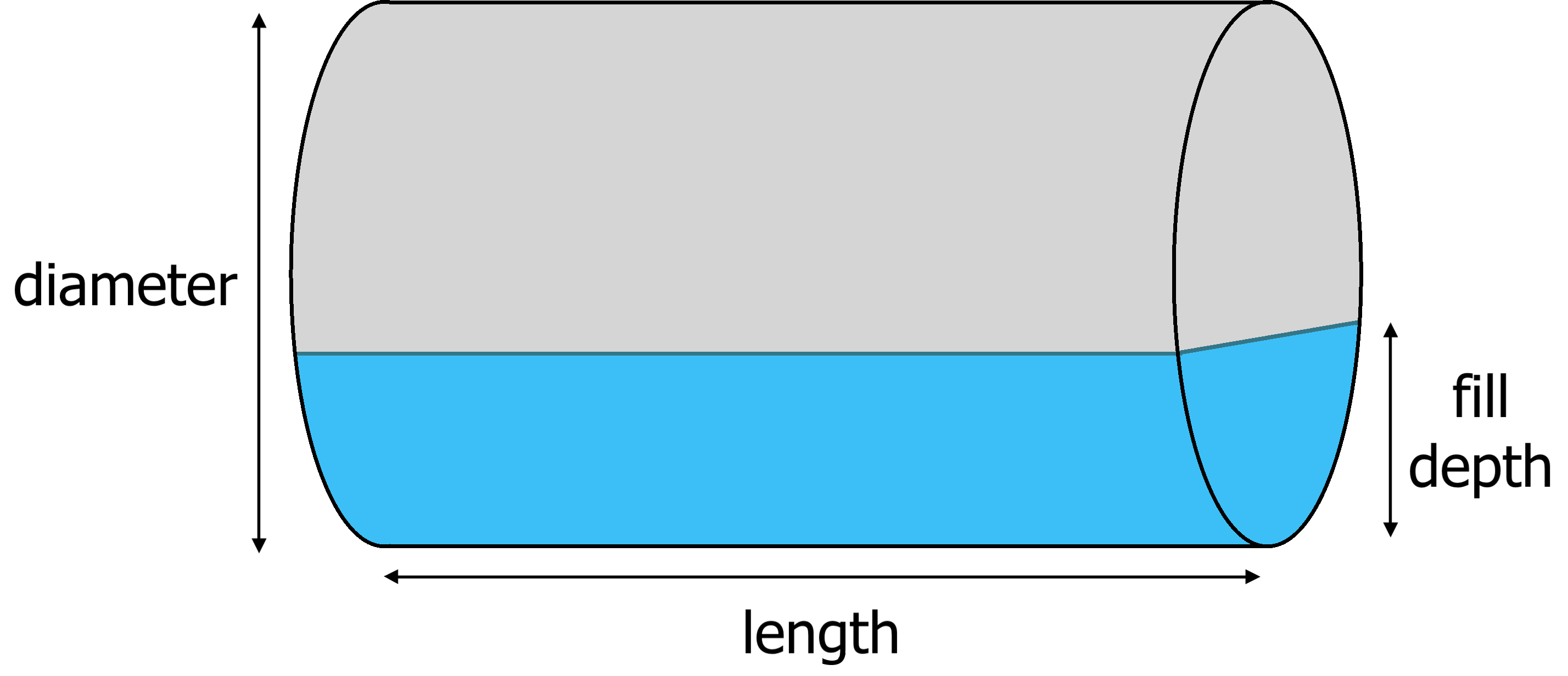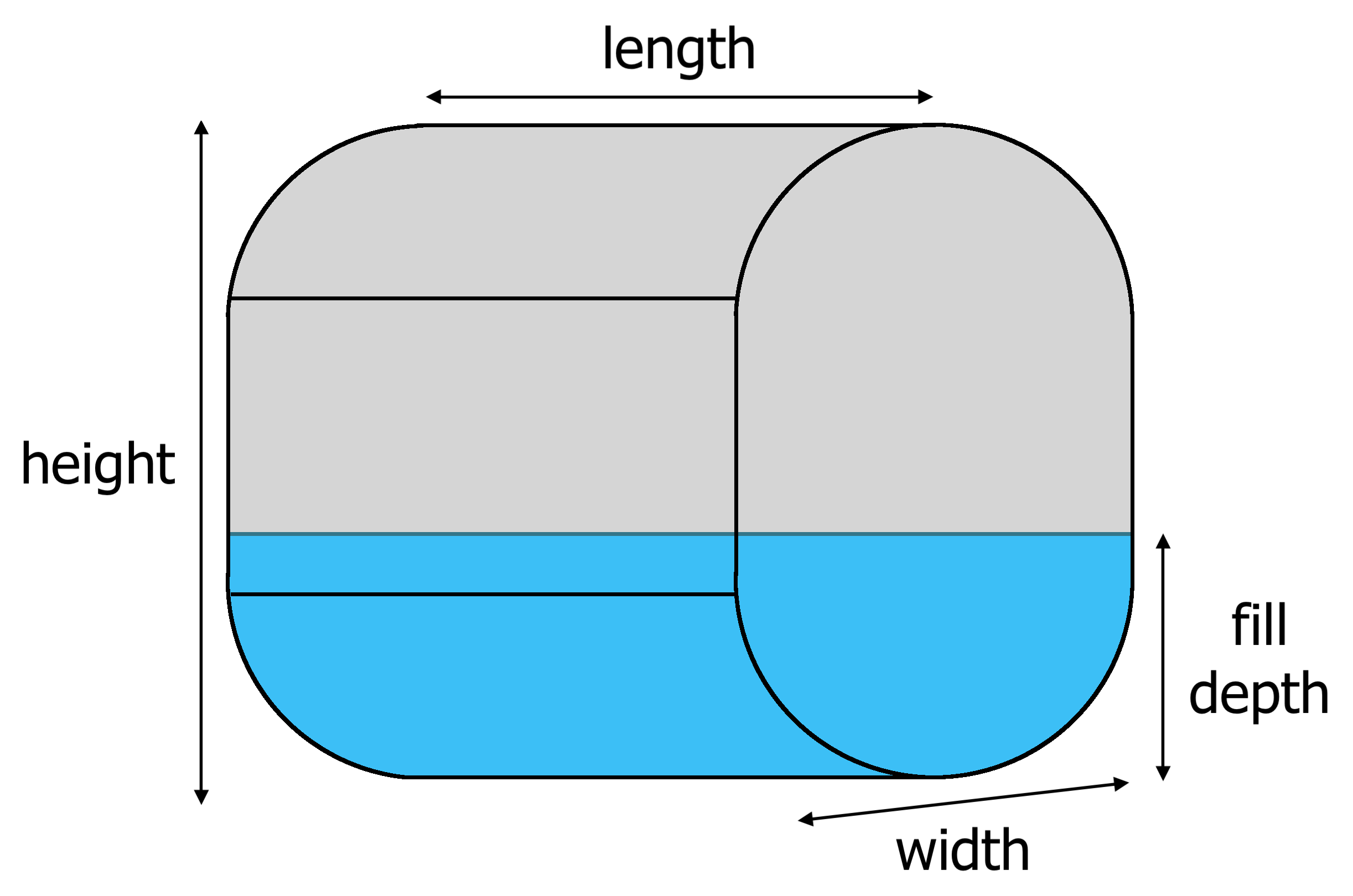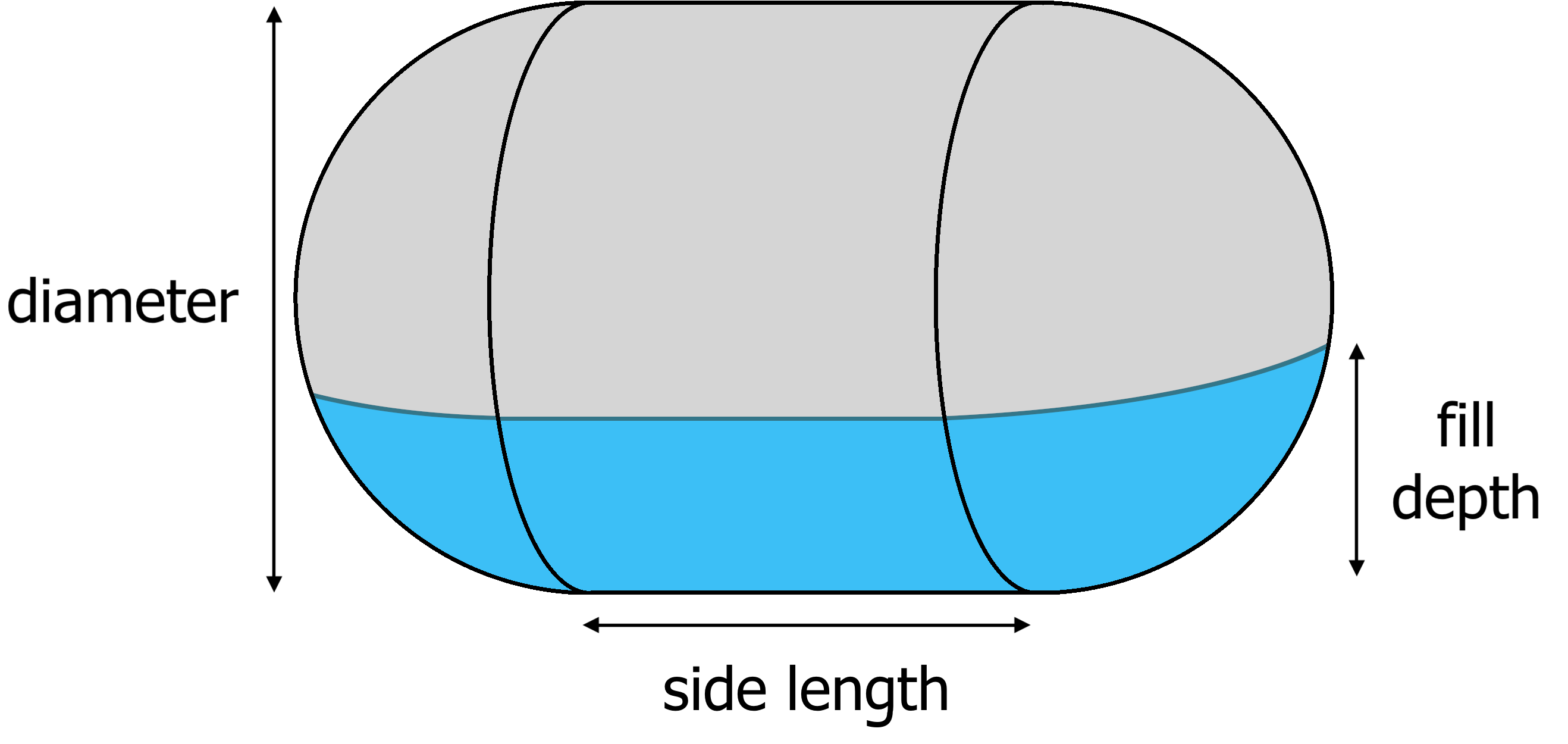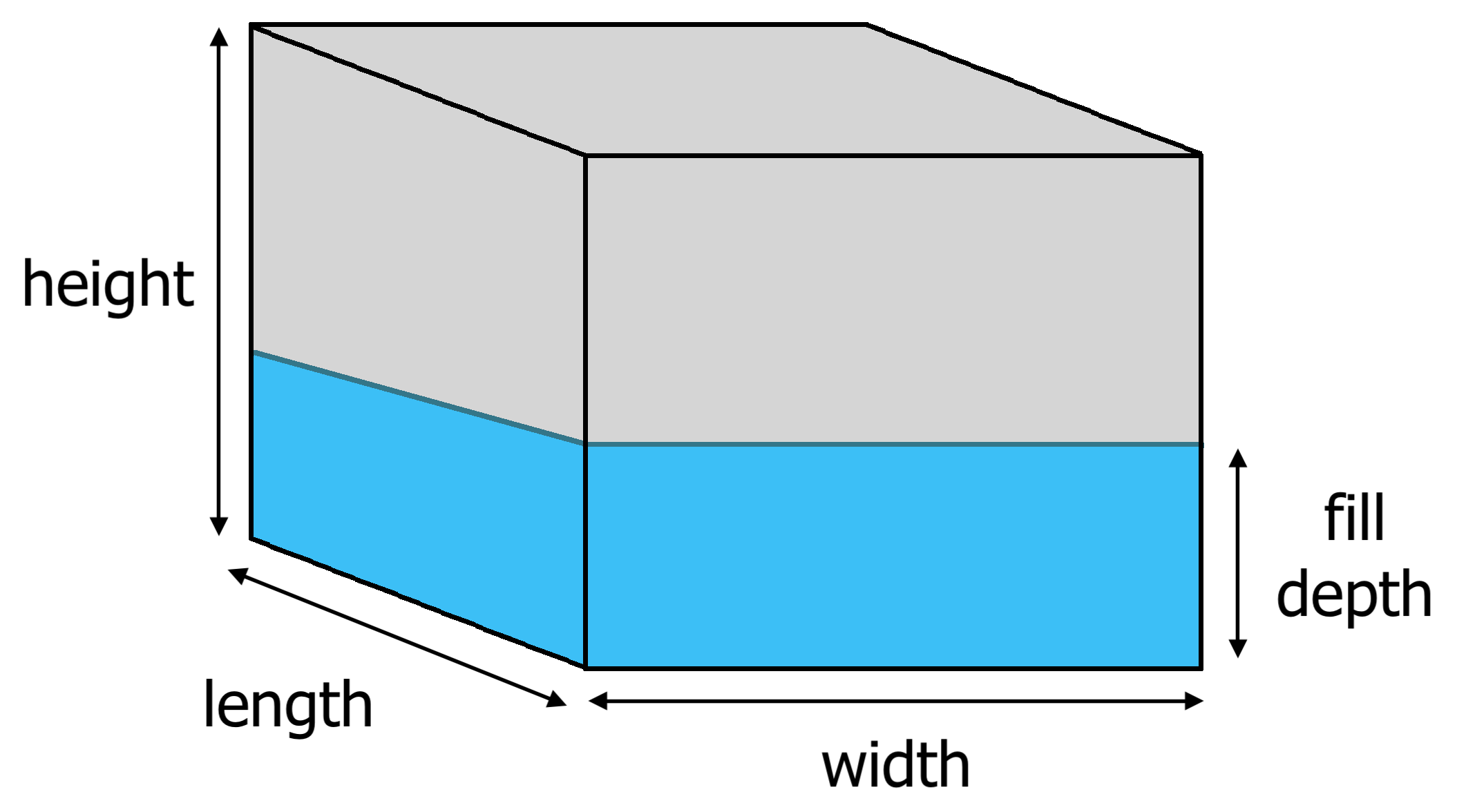# Tank Volume Calculator

Select the style of tank and its dimensions to calculate the capacity. Optionally, enter the filled depth to calculate the volume of liquid in a tank.

Optional

Optional

Optional

Optional

Optional

Optional

Optional

Optional

gallons
liters

gallons
liters

## How to Calculate The Volume of a Tank

The volume or capacity of a tank can be found in a few easy steps. Of course, the calculator above is the easiest way to calculate tank volume, but follow along to learn how to calculate it yourself.

### Step One: Measure the Tank

The first step is to measure the key dimensions of the tank. For round tanks find the diameter and length or height. For rectangular or cubes, find the length, width, and height.

### Step Two: Find the Tank Volume Formula

In order to calculate a tank’s capacity a formula for volume will be needed. The volume formula varies by the style of tank being measured. See the formulas below and select the one for your style.

#### Cylinder Tank Formula

tank volume = π × r2 × l

r = radius (diameter ÷ 2)
l = length (or height for a vertical tank)#### Oval Tank Formula

area = ((h – w) × w) + (π × r2)
tank volume = area × l

r = radius (tank width ÷ 2)
w = width
l = length
h = height#### Capsule Tank Formula

cylinder volume = π × r2 × l
sphere volume = 4/3 π × r3
tank volume = cylinder volume + sphere volume

r = radius (diameter ÷ 2)
l = length (or height for a vertical tank)#### Rectangular Tank Formula

tank volume = l × w × h

l = length
w = width
h = heightNot finding a formula for the shape of your tank? Find even more volume formulas.

### Step Three: Solve the Volume Formula

When you have the tank dimensions and the appropriate formula to solve for volume, simply enter the dimensions into the formula and solve.

For example, let’s find the volume of a cylinder tank that is 36″ in diameter and 72″ long.